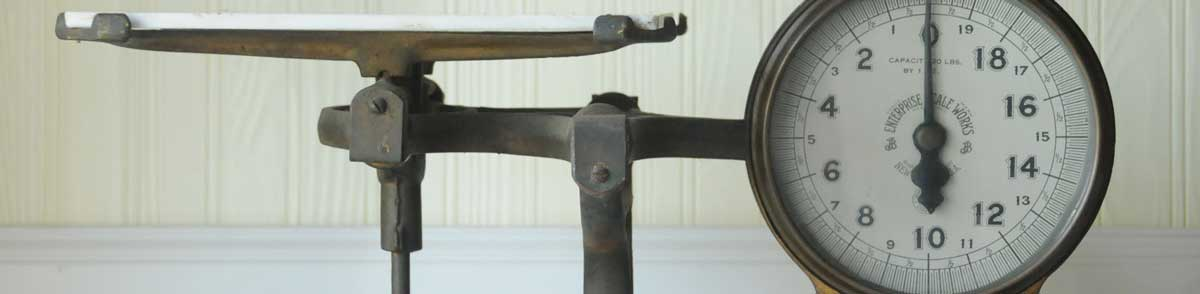# Ounces to Tons Conversion

Enter the weight in ounces below to get the value converted to tons.

Results in Tons:1 oz = 3.125E-5 t
Hint: use a scientific notation calculator to convert E notation to decimal
Do you want to convert tons to ounces?

## How to Convert Ounces to TonsTo convert an ounce measurement to a ton measurement, divide the weight by the conversion ratio.

Since one ton is equal to 32,000 ounces, you can use this simple formula to convert:

tons = ounces ÷ 32,000

The weight in tons is equal to the ounces divided by 32,000.

For example, here's how to convert 50,000 ounces to tons using the formula above.
50,000 oz = (50,000 ÷ 32,000) = 1.5625 t

## Ounces

One ounce is a unit of mass/weight equal to 1/16 of a pound. The common ounce should not be confused with the troy ounce, which is equal to 1/12 of a troy pound.

The ounce is a US customary and imperial unit of weight. Ounces can be abbreviated as oz; for example, 1 ounce can be written as 1 oz.

## Tons

One short ton, sometimes called a US ton, is equal to 2,000 pounds and is mostly used in the US as a unit of mass/weight. The short ton should not be confused with the long ton, which is used mostly in the United Kingdom, or the metric ton which is used in most other countries.

The ton is a US customary unit of weight. A ton is sometimes also referred to as a short ton. Tons can be abbreviated as t; for example, 1 ton can be written as 1 t.

## Ounce to Ton Conversion Table

Ounce measurements converted to tons
Ounces Tons
1 oz 0.00003125 t
2 oz 0.0000625 t
3 oz 0.00009375 t
4 oz 0.000125 t
5 oz 0.000156 t
6 oz 0.000188 t
7 oz 0.000219 t
8 oz 0.00025 t
9 oz 0.000281 t
10 oz 0.000313 t
100 oz 0.003125 t
1,000 oz 0.03125 t
10,000 oz 0.3125 t
100,000 oz 3.125 t

## References

1. James Bucki, Troy Ounce vs. Ounce, The Spruce, https://www.thesprucecrafts.com/troy-ounce-vs-ounce-4588158
2. United States Department of Agriculture, Weights, Measures, and Conversion Factors for Agricultural Commodities and Their Products, https://www.ers.usda.gov/webdocs/publications/41880/33132_ah697_002.pdf